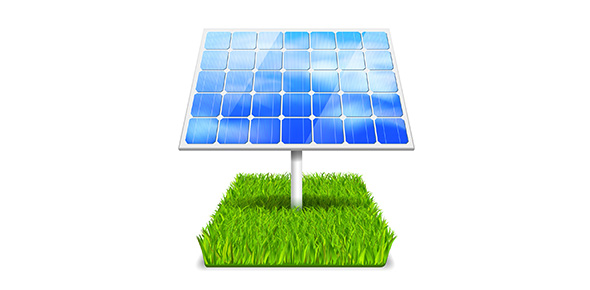# What Do You Know About Energy?

37 Questions | Attempts: 78
ShareSettings• 1.
Potential energy is stored energy
• A.

True

• B.

False

• 2.
Kinetic energy is stored energy
• A.

True

• B.

False

• 3.
Energy efficiency
• A.

The total amount of useful energy

• B.

Useful energy/ total energy

• C.

Is the same as the Law of Conservation of Energy

• D.

Total energy/useful energy

• 4.
All are examples of potential energy except
• A.

Gasoline

• B.

A moving bicycle

• C.

Food

• D.

A battery

• 5.
Which of the following is a conductor
• A.

Wood

• B.

Copper

• C.

Plastic

• D.

Styrofoam

• 6.
Why use styrofoam to hold a cold soda?
• A.

It's a good conductor, therefore the soda will stay cold

• B.

It's a good insulator, therefore heat moves into the soda slowly

• C.

It's a good insulator, therefore cold moves out of the soda slowly

• D.

• 7.
Movement of electricity from Mr. Burns power plant to our house is an example of energy transformation
• A.

True

• B.

False

• 8.
Electricity is potential energy
• A.

True

• B.

False

• 9.
The total amount of energy in the universe is _______
• 10.
Temperature is a measure of the average amount of _______ energy in a substance
• 11.
Gravitational potential energy is greater in Iowa City than in Denver
• A.

True

• B.

False

• 12.
Heat moves from colder areas to warmer areas
• A.

True

• B.

False

• 13.
A pot of boiling water will make a room warmer
• A.

True

• B.

False

• 14.
Ted's hand touches a can of cold soda.  What happens?
• A.

Heat moves in both directions

• B.

No movement of heat occurs

• C.

Heat moves from Ted's hand to the can

• D.

Heat moves from the can to Ted's hand

• 15.
Atoms in a cold soda move _______ than the atoms in a warm soda.
• 16.
Nuclear energy in the sun is  ____________ into chemical energy in plants
• 17.
Chemical energy in plants is _______ into chemical energy in our body.
• 18.
Chemical energy in our body is _________ into mechanical energy when we shot a 3 pt shot.
• 19.
When Stephen Goskowski kicks a field goal, what happens?
• A.

Mechanical energy is transformed into chemical energy

• B.

Chemical energy is transformed into mechanical energy

• C.

Chemical energy is transferred into mechanical energy

• D.

Nuclear energy is transformed into mechanical energy

• 20.
What is the difference between heat and thermal energy
• A.

Thermal energy is in an object, heat is the movement of thermal energy

• B.

No difference, they are the same

• C.

Thermal energy in the movement of heat

• D.

There is more heat energy in the universe

• 21.
The amount of thermal energy in the universe remains constant
• A.

True

• B.

False

• 22.
How efficient is a car that uses 100 units of gasoline to produce 10 units of motion energy?
• A.

1%

• B.

10%

• C.

50%

• D.

100%

• 23.
Frying pans are made from metal but have plastic handle? Why?
• A.

Plastic is a good conductor

• B.

The weights less

• C.

Plastic is a good insulator

• D.

Plastic is a good conductor, making it easier to cook food

• 24.
Sound is a type of potential energy
• A.

True

• B.

False

• 25.
All of the chemical energy from gasoline is converted to mechanical energy.
• A.

True

• B.

False

## Related TopicsBack to top
×

Wait!
Here's an interesting quiz for you.